In this work, the authors demonstrate how CNNs can be used to estimate the orientation of an object between 0o and 360o. For this, they propose and test 3 different methods. For each method, they assume that each image comes with a single prominent object located at the center of the image. Each method is tested with a ResNet-201 pretrained on ImageNet.

# Method 1

Orientation is defined by a vector $$\vec v=(cos(\theta),sin(\theta))$$ and the loss is a L1 norm. During testing, the predicted vector $$\vec v=(x,y)$$ is converted to an angle with a atan2 function.

# Method 2

Same as Method 1 but with a cosine loss $$L(\vec v_{gt},\vec v)=1-cos(\theta)$$ where theta is the angle between the predicted vector and the groundtruth vector.

# Method 3

The third method uses a finite number of discreet $$N=4$$ orientations. In order to reduce the discretization error, they train 3 different networks with different starting orientation angle as shown in fig.2. The softmax prediction of the 3 models are then combined with a mean-shift method and the orientation with the maximum probability is retained.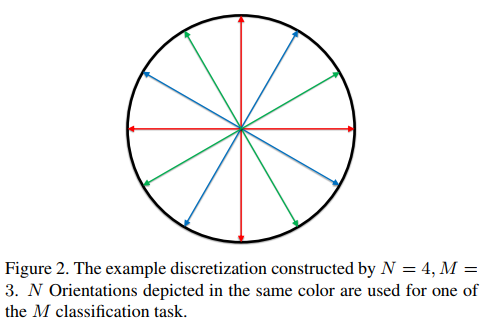# Results

They tested their method on 2 datasets : EPFL-Car and TUD-Pedestrian. In both cases, Method 3 beats Method 1 and 2 as well as previous works.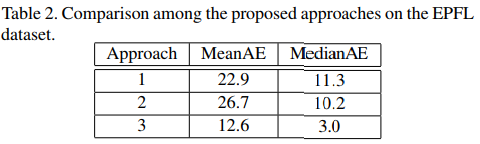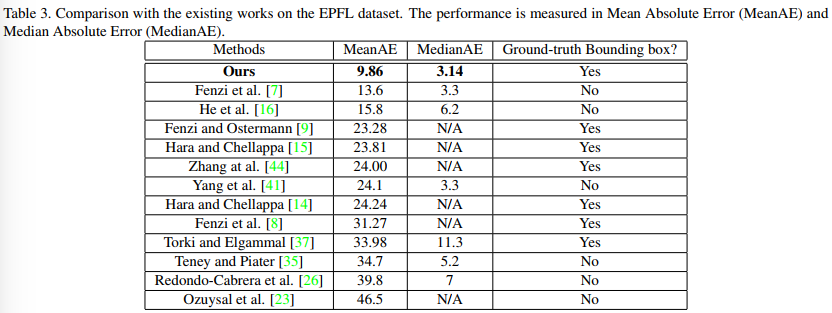Example of results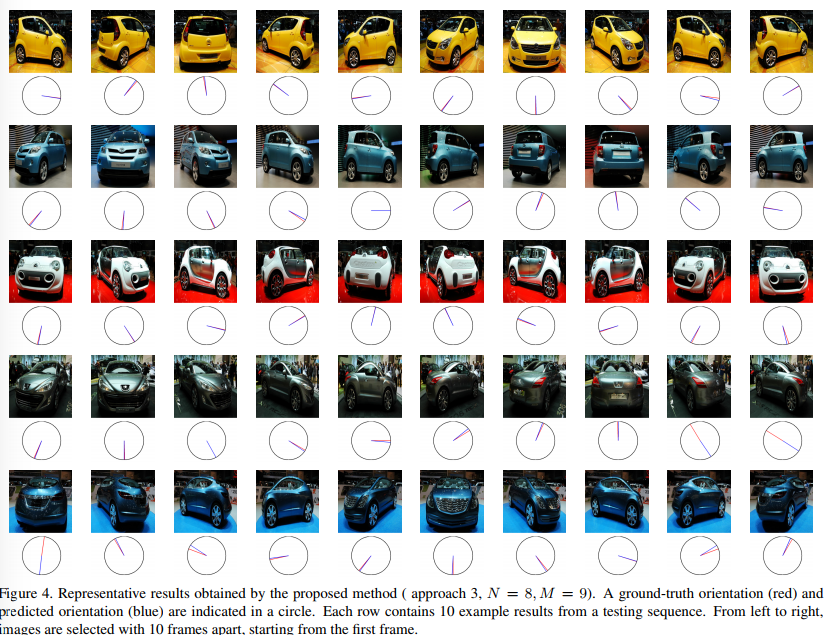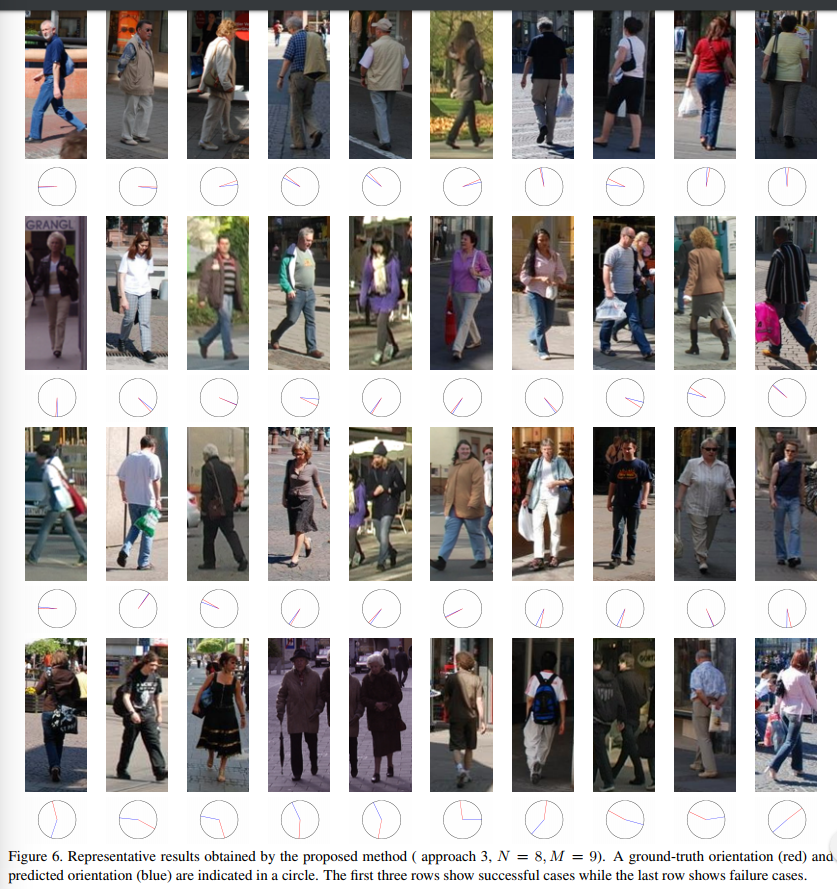# Code

Code is available here